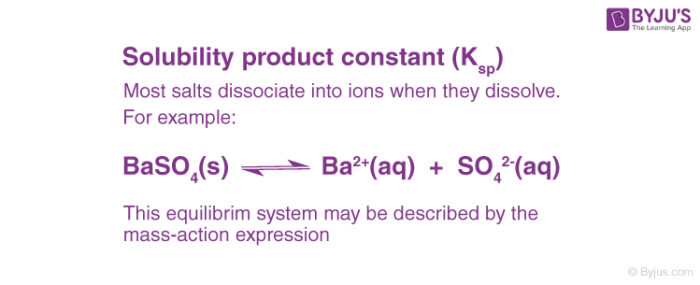# Solubility Product (Ksp)

## What is Solubility Product, Ksp?

The solubility product constant is the equilibrium constant for the dissolution of a solid substance into an aqueous solution. It is denoted by the symbol Ksp.

The solubility product is a kind of equilibrium constant and its value depends on temperature. Ksp usually increases with an increase in temperature due to increased solubility.

Solubility is defined as a property of a substance called solute to get dissolved in a solvent in order to form a solution. The solubility of ionic compounds (which disassociate to form cations and anions) in water varies to a great deal. Some compounds are highly soluble and may even absorb moisture from the atmosphere whereas others are highly insoluble.

## Significance of Solubility Product

Solubility depends on a number of parameters amongst which lattice enthalpy of salt and solvation enthalpy of ions in the solution are of most importance.• When a salt is dissolved in a solvent the strong forces of attraction of solute (lattice enthalpy of its ions) must be overcome by the interactions between ions and the solvent.
• The solvation enthalpy of ions is always negative which means that energy is released during this process.
• The nature of the solvent determines the amount of energy released during solvation that is solvation enthalpy.
• Non-polar solvents have a small value of solvation enthalpy, meaning that this energy is not sufficient to overcome the lattice enthalpy.
• So the salts are not dissolved in non-polar solvents. Hence, for salt to be dissolved in a solvent, its solvation enthalpy should be greater than its lattice enthalpy.
• Solubility depends on temperature and it is different for every salt.

Salts are classified on the basis of their solubility in the following table.

 Category I Soluble Solubility > 0.1M Category II Slightly soluble 0.01M< Solubility<0.1M Category III Sparingly soluble Solubility < 0.1M

## Solubility Product Constant

Suppose barium sulphate along with its saturated aqueous solution is taken. The following equation represents the equilibrium set up between the undissolved solids and ions:

$BaSO_4 \overset{saturated ~solution ~in~ water}{\rightleftharpoons} Ba^{2+} (aq) + SO_4^{-} (aq)$

The equilibrium constant in the above case is:

$K$ = $\frac{[Ba^{2+}][SO_4^{-}]}{[BaSO_{4}]}$

In case of pure solid substances the concentration remains constant, and so we can say:

$K_{sp}$ = $K[BaSO_4]$ = $[Ba^{2+}][SO_4^{-}]$

Here $K_{sp}$ is known as the solubility product constant. This further tells us that solid barium sulphate when in equilibrium with its saturated solution, the product of concentrations of ions of both barium and sulphate is equal to the solubility product constant.

## Related VideosWe know have a brief idea about the solubility of a compound and the factors affecting it. For any further query on this topic, call the mentor support team at BYJU’S.

## Frequently Asked Questions – FAQs

### What is the difference between solubility and solubility product constant?

The solubility of a substance in a solvent is the total amount of the solute that can be dissolved in the solvent at equilibrium. On the other hand, the solubility product constant is an equilibrium constant that provides insight into the equilibrium between the solid solute and its constituent ions that are dissociated across the solution.

### What is the expression for the solubility product constant of magnesium fluoride?

When dissolved in polar solvents, one magnesium fluoride molecule dissociates into one magnesium cation and two fluoride anions. This equilibrium reaction can be represented as follows.

MgF2 ⇌ Mg2+ + 2F

Therefore, the solubility product constant can be expressed as:

Ksp = [Mg2+][F]2

### What is the expression for the solubility product constant of common salt?

The chemical formula of common salt is NaCl. When dissolved in polar solvents, one sodium chloride molecule dissociates into one sodium cation and one chloride anion. This equilibrium reaction can be represented as follows.

NaCl ⇌ Na+ + Cl

Therefore, the solubility product constant can be expressed as:

Ksp = [Na+][Cl]

### What is the solubility product constant for calcium chloride?

The chemical formula of calcium chloride is CaCl2. When dissolved in polar solvents, one calcium chloride molecule dissociates into one calcium cation and two chloride anions. This equilibrium reaction can be represented as follows.

CaCl2 ⇌ Ca2+ + 2Cl

Therefore, the solubility product constant can be expressed as:

Ksp = [Ca2+][Cl]2

### What factors affect the value of Ksp?

Some important factors that have an impact on the solubility product constant are:

• The common-ion effect (the presence of a common ion lowers the value of Ksp).
• The diverse-ion effect (if the ions of the solutes are uncommon, the value of Ksp will be high).
• The presence of ion-pairs.

Take up a quiz on solubility-product-constant

#### 1 Comment

1. Kato Ismail

Good content I think
Thanks alot